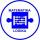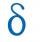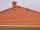# Sequence 3

Write the first 5 members of an arithmetic sequence: a4=-35, a11=-105.

Result

a1 =  -5
a2 =  -15
a3 =  -25
a4 =  -35
a5 =  -45

#### Solution:Leave us a comment of example and its solution (i.e. if it is still somewhat unclear...):Be the first to comment!## Next similar examples:

1. SequenceWrite the first 6 members of these sequence: a1 = 5 a2 = 7 an+2 = an+1 +2 an
2. Sequence 2Write the first 5 members of an arithmetic sequence a11=-14, d=-1
3. SequenceWrite the first 7 members of an arithmetic sequence: a1=-3, d=6.
4. AS - sequenceWhat are the first ten members of the sequence if a11=22, d=2.
5. AP - basicsDetermine first member and differentiate of the the following sequence: a3-a5=24 a4-2a5=61
6. AP - simpleDetermine the first nine elements of sequence if a10 = -1 and d = 4
7. Six termsFind the first six terms of the sequence a1 = -3, an = 2 * an-1
8. AS sequenceIn an arithmetic sequence is given the difference d = -3 and a71 = 455. a) Determine the value of a62 b) Determine the sum of 71 members.
9. SequenceBetween numbers 1 and 53 insert n members of the arithmetic sequence that its sum is 702.
10. Nineteenth memberFind the nineteenth member of the arithmetic sequence: a1=33 d=5 find a19
11. Difference APCalculate the difference of arithmetic progression if the sum of its first 19 members Sn = 8075 and the first member is a1 = 20
12. SeatsSeats in the sport hall are organized so that each subsequent row has five more seats. First has 10 seats. How many seats are: a) in the eighth row b) in the eighteenth row
13. Third memberDetermine the third member of the AP if a4=93, d=7.5.
14. RoofTiles are stacked in rows on the trapezoidal shaped roof. At the ridge is 15 tiles and each subsequent row has one more tile than in the previous row. How many tiled is covered roof if lowermost row has 37 tiles?
15. Difference AP 4Calculate the difference of the AP if a1 = 0.5, a2 + a3 = -1.1
16. Theorem proveWe want to prove the sentence: If the natural number n is divisible by six, then n is divisible by three. From what assumption we started?
17. Calculation of CNCalculate: ?